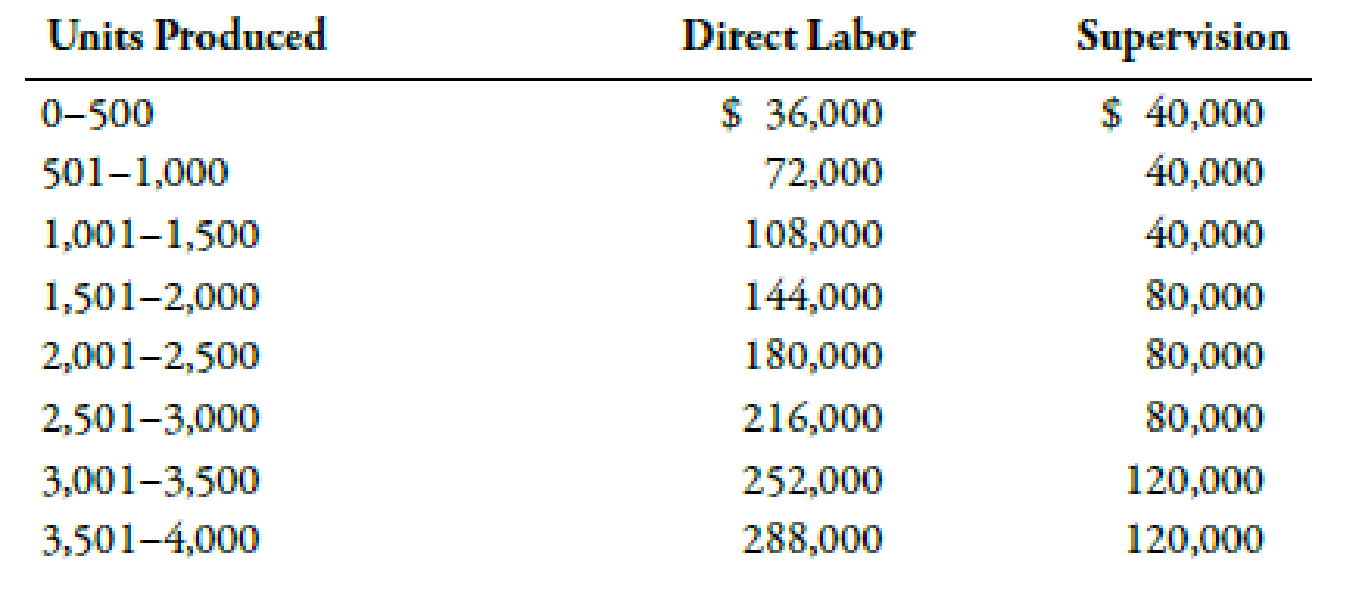Chapter 3, Problem 39E### Managerial Accounting: The Corners...

7th Edition
Maryanne M. Mowen + 2 others
ISBN: 9781337115773

#### Solutions

Chapter
Section### Managerial Accounting: The Corners...

7th Edition
Maryanne M. Mowen + 2 others
ISBN: 9781337115773
Textbook Problem
1 views

# Step Costs, Relevant RangeBellati Inc. produces large industrial machinery. Bellati has a machining department and a group of direct laborers called machinists. Each machinist can machine up to 500 units per year. Bellati also hires supervisors to develop machine specification plans and oversee production within the machining department. Given the planning and supervisory work, a supervisor can oversee, at most, three machinists. Bellati’s accounting and production history shows the following relationships between number of units produced and the annual costs of supervision and materials handling (by machinists):Required: 1. Prepare a graph that illustrates the relationship between direct labor cost and number of units produced in the machining department. (Let cost of direct labor be the vertical axis and number of units be the horizontal axis.) Would you classify this cost as a strictly variable cost, a fixed cost, or a step cost? 2. Prepare a graph that illustrates the relationship between the cost of supervision and the number of units produced. (Let cost of supervision be the vertical axis and number of units be the horizontal axis.) Would you classify this cost as a strictly variable cost, a fixed cost, or a step cost? 3. Suppose that the normal range of production is between 1,400 and 1,500 units and that the exact number of machinists is currently hired to support this level of activity. Further suppose that production for the next year is expected to increase by an additional 500 units. What is the increase in the cost of direct labor? Cost of supervision?

1.

To determine

Construct the graph that explains the relationship between direct labor cost and number of units produced in the machining department. Also, identify this type of cost as a variable cost, a fixed cost, or step cost.

Explanation

Cost:

Cost can be defined as the cash and cash equivalent which is incurred against the products or its related services which will benefit the organization in the future. There are two types of costs that are fixed and variable costs.

2.

To determine

Construct the graph that explains the relationship between the cost of supervision and number of units produced in the machining department. Also, identify this type of cost as a variable cost, a fixed cost, or step cost.

3.

To determine

Compute the amount of increase in direct labor cost. Also, compute the amount of cost of supervision.

### Still sussing out bartleby?

Check out a sample textbook solution.

See a sample solution

#### The Solution to Your Study Problems

Bartleby provides explanations to thousands of textbook problems written by our experts, many with advanced degrees!

Get Started